# Pythagoras' Theorem Math Review Tutorial

math tutorials > pythagoras' theorem

## Pythagorus' Theorem states:

For any right-angled triangle, the square on the hypotenuse is equal to the sum of the squares on the other 2 sides.

In a right-angled triangle, the side opposite the right angle is called the hypotenuse.

## Step 1

So Pythagorus' Theorem could be shown like this...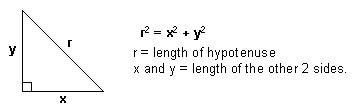We can use this formula to calculate the length of an unknown side in a right angle triangle.

### Example

Calculate x: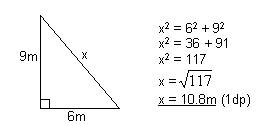## Pythagoras problems for you to try

Calculate the unknown sides.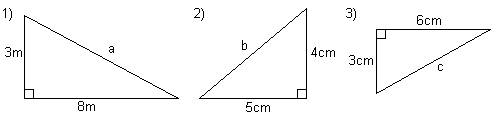Solutions:  1)  8.5m (1dp)    2)  6.4cm (1dp)     3)  6.7cm (1dp)

## Step 2

When you are given the length of the hypotenuse and are required to calculate the length of one of the other sides in a right-angled triangle, you need to rearrange the Pythagorus' Theorem.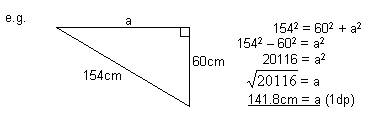## Pythagoras problems for you to try

Calculate the length of the unknown side.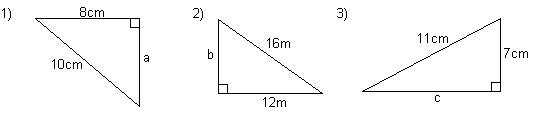Solutions:  1)  6cm     2)  10.6m (1dp)     3)  8.5cm (1dp)